# Determine Compressibility of Gases

This article will demonstrate how to determine gas compressibility by using simplified equation of state.

At a low pressure condition, gas similarly behaves like ideal gas and the equation for the ideal gas law is listed below;

# PV = nRT

Where;

P = pressure

V = gas volume

T = absolute temperature

n = number of moles of gas

R = gas constant

Gas constant (R) is different because it depends on the unit system used in the calculation. The R in the different unit system is demonstrated in the below table.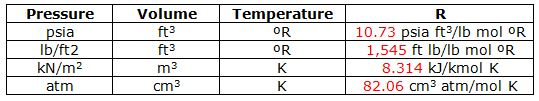Table 1 – Gas Constant (R) at Different Unit

At normal pressure and temperature, the ideal gas law is accurate to determines gas behavior. However, the ideal gas law cannot be applied to gas in a reservoir as pressure and temperature in a reservoir is much higher than atmospheric conditions. Therefore, the real gas equation has a compressibility factor(z) to correct the real gas relationship. The real gas relationship can be shown below;

# PV = znRT

Where;

P = pressure

V = gas volume

T = absolute temperature

n = number of moles of gas

R = gas constant

z = compressibility factor

z=1.0 means the ideal gas.

The compressibility factor (z) is changed by temperature and pressure. The two charts in Figure 1 demonstrate how z-factor changes with temperature and pressure.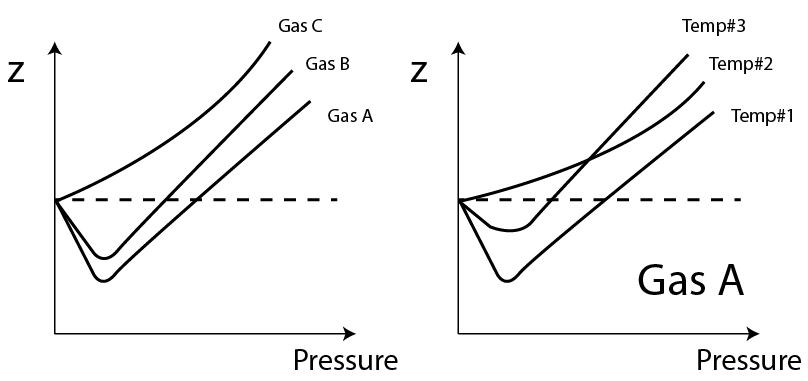Figure 1– Compressibility factor at different pressure and temperature

At a reservoir condition, gas is mixtures of several gas molecules therefore it is impossible to have the z-factor chart made to match with each composition of gas in a reservoir. Therefore, the Principle of Corresponding States by Van der Waals (in 1873) is utilized to describe gas in reservoir conditions. The concept of the Principle of Corresponding States proposes that the equation state shown in reduced gas properties is the same for all gas types and mixtures.

The reduce properties are shown below;

PR (reduced pressure) = P ÷ Pc

VR (reduced volume) = V ÷ Vc

TR (reduced temperature) = T ÷ Tc

Where;

P = current pressure

Pc= critical pressure

V = current volume

Vc = critical volume

T = current temperature

Tc = critical temperature

Note: the critical point is where the liquid and the vapor have the same properties.

Critical Pressure and Temperature Gas in Imperial Unit is shown in Figure 2.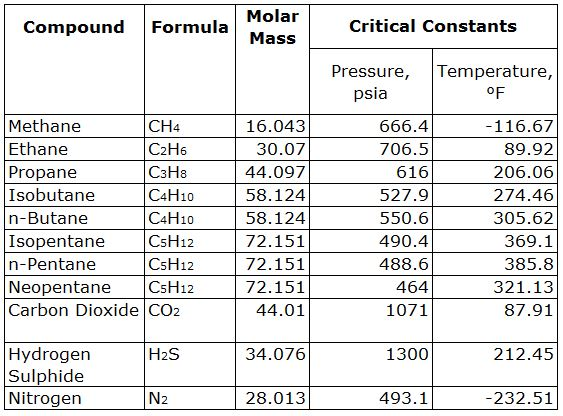Table 2 – Critical Pressure and Temperature of Gas in Imperial Unit

Critical Pressure and Temperature Gas in SI Unit is shown in Table 3.Table 3 – Critical Pressure and Temperature of Gas in SI Unit

How To Determine z-Factor of Mixtures of Gas

The Standing and Katz (1941) chart is widely used to estimate reservoir gas compressibility factor (z). The z-factor chart is shown below. The x-axis is pseudo reduced pressure and  each line represents pseudo reduce temperature. The y-axis is the compressibility factor (z).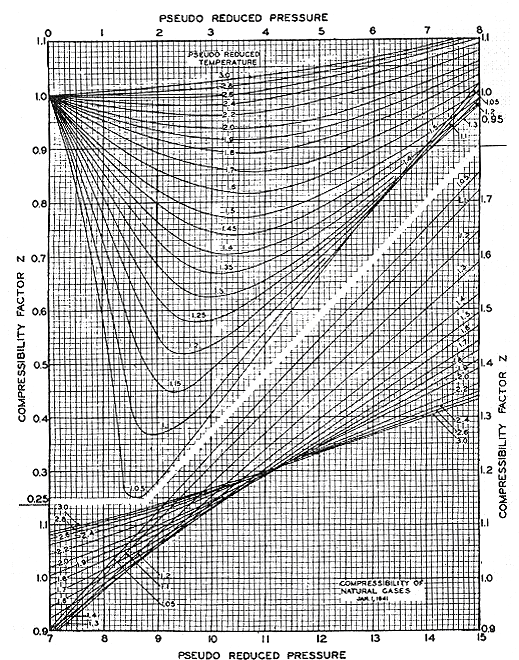Figure 2 – The Standing and Katz (1941) chart

The pseudo-reduced properties are used in order to deal with all components of gas and the relationships are expressed below;

PR’ (pseudo reduced pressure) = P ÷ Pc’

TR’ (pseudo reduced temperature) = T ÷ Tc’

Where;

PR’ = pseudo-reduced pressure

TR’ = pseudo-reduced temperature

P = current pressure

Pc’= pseudo-critical pressure

T = current temperature

Tc’ = pseudo-critical temperature

Pseudo-critical Temperature and Pressure can be calculated by a summation of weighted average of critical temperature and pressure of each component (Kay’s Rules).

Pc’ = y1Pc1 + y2Pc2 + y3Pc3 + y4Pc4 +…

Tc’ = y1Tc1 + y2Tc2 + y3Tc3 + y4PTc4 +…

Steps to Determine z-Factor Based on Pseudo-Properties

1. Determine pseudo-critical pressure (Pc’ ) and temperature (Tc’)
2. Determine pseudo-reduce pressure (PR’) and temperature (TR’)
3. Utilize the Standing and Katz (1941) chart (Figure 2) to get the value of z-factor from the chart.

Correction for Acid Gases

If acid gases as carbon dioxide and/or hydrogen sulphide are more than 5% by volume, the correction must be applied. The Wichert and Aziz correction is used to correct the effect of acid gas contents.

The correction factor (ɛ) that is used to correct the pseudo-critical temperature and pressure is given by: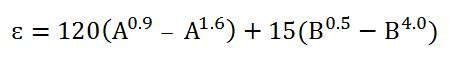Where

A =sum of mole fractions of carbon dioxide (CO2) and hydrogen sulphide (H2S) in the mixture

B = mole fraction of hydrogen sulphide (H2S) in the mixture.

Once the correction factor (ɛ) is determined, the corrected pseudo-critical temperature and pressure can be calculated by the following equations.

Corrected pseudo-critical temperature (Tc”)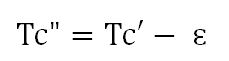Corrected pseudo-critical pressure (Pc”)Where;

Pc’= pseudo-critical pressure

Tc’ = pseudo-critical temperature

After calculating the corrected pseudo-critical temperature (Tc”) and corrected pseudo-critical pressure (Pc”), use these figures to calculate the corrected pseudo-reduced pressure and temperature. Then use the Standing and Katz chart to obtain a compressibility factor (z) in a normal way.

References

Abhijit Y. Dandekar, 2013. Petroleum Reservoir Rock and Fluid Properties, Second Edition. 2 Edition. CRC Press.

L.P. Dake, 1983. Fundamentals of Reservoir Engineering, Volume 8 (Developments in Petroleum Science). New impression Edition. Elsevier Science.

Tarek Ahmed PhD PE, 2011. Advanced Reservoir Management and Engineering, Second Edition. 2 Edition. Gulf Professional Publishing.

Share the joy
Tagged , , , . Bookmark the permalink.